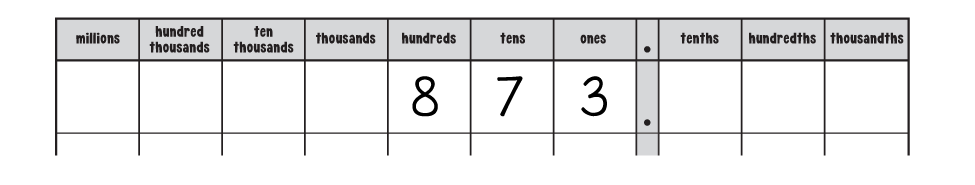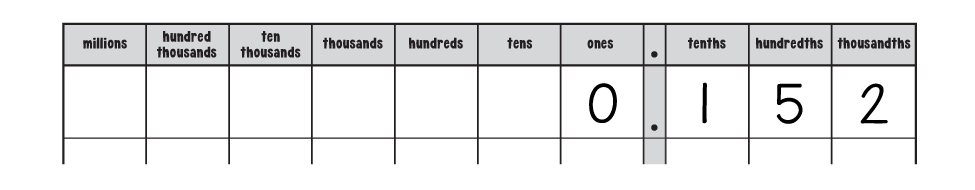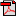# Lesson 5: Comparing Decimals

## Activities

### Activity 1: Using a Decimal Place Value Chart

Materials: fine point dry-erase markers (kit), laminated decimal place value chart (kit), number cards (kit)
Using the laminated decimal place value chart and a dry-erase marker, write 873 in the first row, making sure to place each digit in the correct column, as shown in the following image.Now, write 973 in the second row on the chart. Which of the numbers on the chart, 873 and 973, is greater? You know that 973 is greater because nine hundreds is greater than eight hundreds.
Now, write 843 in the third row on the chart. Which is greater, 873 or 843? You should find that 873 is greater because, when comparing multi-digit numbers, if the digits in the highest places are the same you look to the next highest place and compare those digits.

Guess what? Comparing decimals works in just the same way. Watch the following video for an explanation of how decimal number comparison works.
Now it's your turn. Erase the numbers on the laminated decimal value chart and write 0.152 in the first row.Then, in the next rows, write the following numbers: 0.267 and 0.289. To compare 0.152 and 0.267, begin by looking at the highest place. In this case, it's the ones place, but both numbers have zero in the ones place. So, next, look at the tenths place. Which number has more tenths? That is the greater of the two numbers. You should find that 0.267 is greater than 0.152.

Next, compare 0.267 and 0.289. Which is greater? You should find that 0.289 is greater because both numbers have 2 in the tenths place but the 8 in the hundredths place in 0.289 is more than the 6 in the hundredths place in 0.267.

Now, erase the numbers on the laminated decimal place value chart. Write 0 in each row in the ones column. Using number cards 1 through 9, shuffle the cards, and place them face down. Draw 2 cards, and use the numbers showing to create a decimal to the hundredths place. Write this number in the first row on the chart. For example, if you draw 3 and 8, you can write either 0.38 or 0.83. Repeat this process until the chart is full, reshuffling the cards when you need to.

Use the numbers on the chart to complete the "Comparing Decimals: Hundredths" sheet. When you finish the sheet, share your answers with a parent, and explain the steps in comparing decimals.Student Activity Page
Your child will now use a decimal place value chart to compare decimals by looking at specific digits and places in numbers. Once he completes the "Comparing Decimals: Hundredths" sheet, he will share his answers with you and will explain how to compare decimals. Make sure that his work is correct and that he can identify the steps in comparing decimals: look at the highest place first, and compare the digits there. If those digits are the same, move to the next highest place, and compare the digits there. As needed, remind him that tenths are bigger than hundredths, and hundredths are bigger than thousandths, and encourage him to use the laminated decimal grid if he needs help picturing any of the numbers.

The answers to the questions at the bottom of the sheet are follows:
• Circle the decimal that is less than the one shown: (0.36)
• Circle the decimal that is more than the one shown: (0.50)

### Activity 2: Comparing Thousandths

Complete the "Comparing Decimals: Thousandths" sheet. Use the laminated decimal grid or laminated decimal place value chart as needed to help you with the comparisons.Student Activity Page
Your child will work on comparing decimals to thousandths.